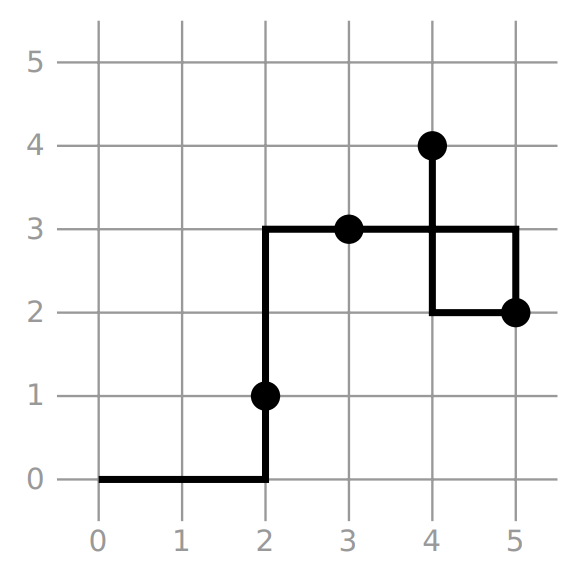시간 제한 메모리 제한 제출 정답 맞은 사람 정답 비율
0.1 초 512 MB 2 2 2 100.000%

## 문제

Azerbaijan is famous for its carpets. As a master carpet designer you want to make a new design by drawing a broken line. A broken line is a sequence of $t$ line segments in a two-dimensional plane, which is defined by a sequence of $t+1$ points $p_0, \ldots, p_t$ as follows. For each $0 \leq j \leq t-1$ there is a segment connecting points $p_j$ and $p_{j+1}$.

In order to make the new design, you have already marked $n$ dots in a two-dimensional plane. The coordinates of dot $i$ ($1 \leq i \leq n$) are $(x[i], y[i])$. No two dots have the same x or the same y coordinate.

You now want to find a sequence of points $(sx, sy), (sx, sy), \ldots, (sx[k], sy[k])$, which defines a broken line that

• starts at $(0, 0)$ (that is, $sx = 0$ and $sy = 0$),
• contains all of the dots (not necessarily as the endpoints of the segments), and
• consists solely of horizontal or vertical segments (two consecutive points defining the broken line have an equal x or y coordinate).

The broken line is allowed to intersect or overlap itself in any way. Formally, each point of the plane may belong to any number of segments of the broken line.

This is an output-only task with partial scoring. You are given $10$ input files specifying the locations of dots. For each input file, you should submit an output file describing a broken line with the required properties. For each output file that describes a valid broken line your score depends on the number of segments in the broken line (see Scoring below).

## 입력

Each input file is in the following format:

• line $1$: $\;\;n$
• line $1+i$ (for $1 \leq i \leq n$): $\;\; x[i] \;\; y[i]$

## 출력

Each output file must be in the following format:

• line $1$: $\;\;k$
• line $1+j$ (for $1 \leq j \leq k$): $\;\; sx[j] \;\; sy[j]$

Note that the second line should contain $sx$ and $sy$ (i.e., the output should not contain $sx$ and $sy$). Each $sx[j]$ and $sy[j]$ should be an integer.

## 제한

•
• $1 \leq n \leq 100\,000$
• $1 \leq x[i], y[i] \leq {10}^9$
• All values of $x[i]$ and $y[i]$ are integers.
• No two dots have the same $x$ or the same $y$ coordinates, i.e. $x[i_1] \neq x[i_2]$ and $y[i_1] \neq y[i_2]$ for $i_1 \neq i_2$.
• $-2 \cdot {10}^9 \leq sx[j], sy[j] \leq 2 \cdot {10}^9$
•

## 예제 입력 1

4
2 1
3 3
4 4
5 2


## 예제 출력 1

6
2 0
2 3
5 3
5 2
4 2
4 4Please note this example is not among the actual inputs of this task.

## 점수

For each test case, you can get up to $10$ points. Your output for a test case will get $0$ points if it does not specify a broken line with the required properties. Otherwise, the score will be determined using a decreasing sequence $c_1, \ldots, c_{10}$, which varies by testcase.

Assume that your solution is a valid broken line consisting of $k$ segments. Then, you will get

• $i$ points, if $k = c_i$ (for $1 \leq i \leq 10$),
• $i + \frac{c_i - k}{c_i - c_{i+1}}$  points, if $c_{i+1} < k < c_i$ (for $1 \leq i \leq 9$),
• $0$ points, if $k > c_1$,
• $10$ points, if $k < c_{10}$.

The sequence $c_1, \ldots, c_{10}$ for each testcase is given below.

Testcases 01 02 03 04 05 06 07-10
$n$ $20$ $600$ $5\,000$ $50\,000$ $72\,018$ $91\,891$ $100\,000$
$c_{1}$ $50$ $1\,200$ $10\,000$ $100\,000$ $144\,036$ $183\,782$ $200\,000$
$c_{2}$ $45$ $937$ $7\,607$ $75\,336$ $108\,430$ $138\,292$ $150\,475$
$c_{3}$ $40$ $674$ $5\,213$ $50\,671$ $72\,824$ $92\,801$ $100\,949$
$c_{4}$ $37$ $651$ $5\,125$ $50\,359$ $72\,446$ $92\,371$ $100\,500$
$c_{5}$ $35$ $640$ $5\,081$ $50\,203$ $72\,257$ $92\,156$ $100\,275$
$c_{6}$ $33$ $628$ $5\,037$ $50\,047$ $72\,067$ $91\,941$ $100\,050$
$c_{7}$ $28$ $616$ $5\,020$ $50\,025$ $72\,044$ $91\,918$ $100\,027$
$c_{8}$ $26$ $610$ $5\,012$ $50\,014$ $72\,033$ $91\,906$ $100\,015$
$c_{9}$ $25$ $607$ $5\,008$ $50\,009$ $72\,027$ $91\,900$ $100\,009$
$c_{10}$ $23$ $603$ $5\,003$ $50\,003$ $72\,021$ $91\,894$ $100\,003$

## 비주얼라이저

In the attachments of this task, there is a script that allows you to visualize input and output files.

To visualize an input file, use the following command:

python vis.py [input file]


You can also visualize your solution for some input, using the following command. Due to technical limitations, the provided visualizer shows only the first $1000$ segments of the output file.

python vis.py [input file] --solution [output file]


Example:

python vis.py examples/00.in --solution examples/00.out

Text

## 채점 및 기타 정보

• 10점 이상을 획득해야 를 받는다.
• 예제는 채점하지 않는다.
• 소스 코드의 크기는 4194304B을 넘을 수 없다.